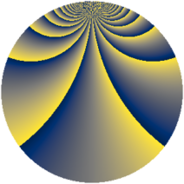# Properties

 Label 2366.2.bnLevel $2366$ Weight $2$ Character orbit 2366.bn Rep. character $\chi_{2366}(83,\cdot)$ Character field $\Q(\zeta_{52})$ Dimension $2976$ Sturm bound $728$

# Related objects

## Defining parameters

 Level: $$N$$ $$=$$ $$2366 = 2 \cdot 7 \cdot 13^{2}$$ Weight: $$k$$ $$=$$ $$2$$ Character orbit: $$[\chi]$$ $$=$$ 2366.bn (of order $$52$$ and degree $$24$$) Character conductor: $$\operatorname{cond}(\chi)$$ $$=$$ $$1183$$ Character field: $$\Q(\zeta_{52})$$ Sturm bound: $$728$$

## Dimensions

The following table gives the dimensions of various subspaces of $$M_{2}(2366, [\chi])$$.

Total New Old
Modular forms 8832 2976 5856
Cusp forms 8640 2976 5664
Eisenstein series 192 0 192

## Trace form

 $$2976q - 8q^{7} + 264q^{9} + O(q^{10})$$ $$2976q - 8q^{7} + 264q^{9} - 16q^{15} + 248q^{16} - 8q^{18} - 4q^{21} - 8q^{28} + 16q^{29} + 104q^{30} - 32q^{35} + 8q^{37} + 144q^{39} - 24q^{42} + 24q^{50} - 32q^{53} - 16q^{57} - 8q^{58} + 16q^{60} + 300q^{63} - 88q^{65} - 320q^{67} - 164q^{70} + 64q^{71} + 8q^{72} - 192q^{74} - 16q^{78} + 48q^{79} - 328q^{81} - 4q^{84} + 264q^{85} + 256q^{86} - 44q^{91} + 16q^{92} - 112q^{93} - 32q^{98} + 16q^{99} + O(q^{100})$$

## Decomposition of $$S_{2}^{\mathrm{new}}(2366, [\chi])$$ into newform subspaces

The newforms in this space have not yet been added to the LMFDB.

## Decomposition of $$S_{2}^{\mathrm{old}}(2366, [\chi])$$ into lower level spaces

$$S_{2}^{\mathrm{old}}(2366, [\chi]) \cong$$ $$S_{2}^{\mathrm{new}}(1183, [\chi])$$$$^{\oplus 2}$$